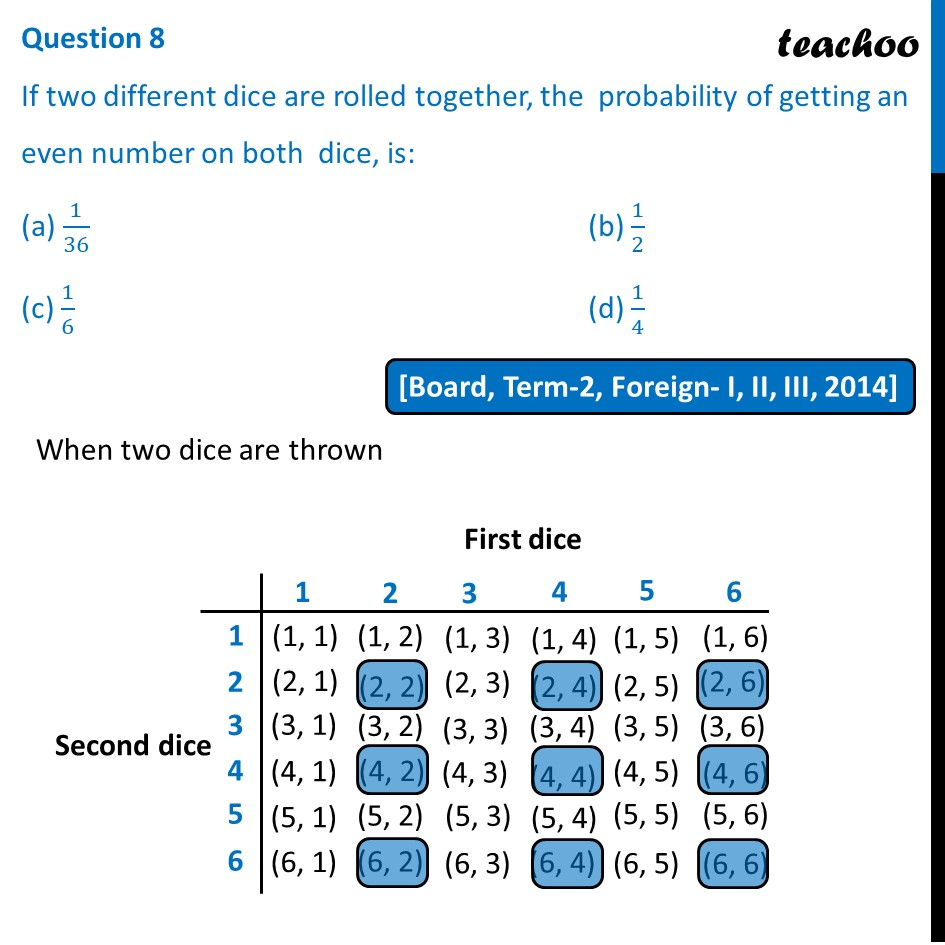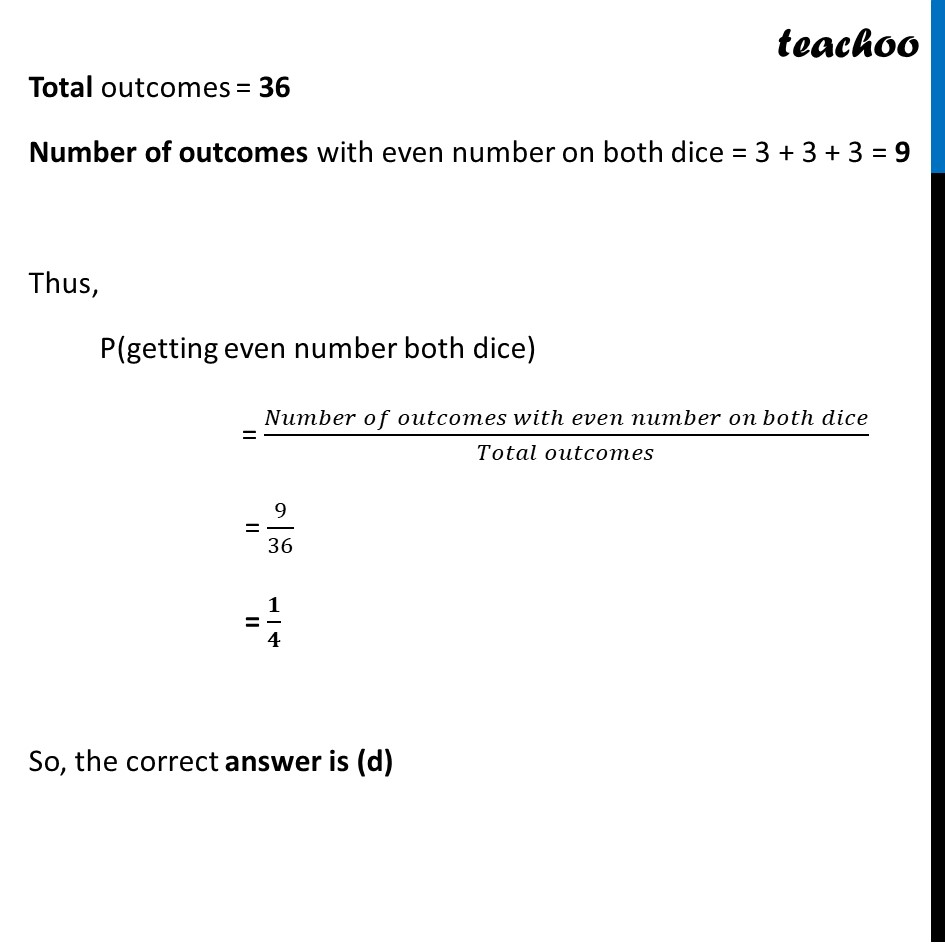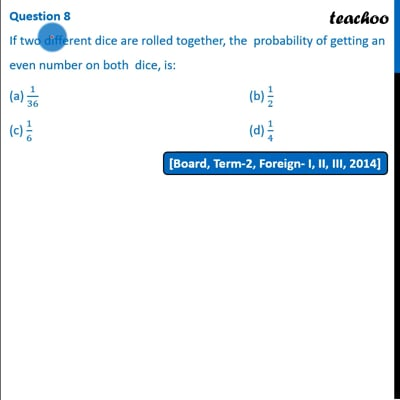Past Year MCQ

Chapter 15 Class 10 Probability
Serial order wise

## (c) 1/6                                                          (d) 1/4This video is only available for Teachoo black users

Maths Crash Course - Live lectures + all videos + Real time Doubt solving!

### Transcript

Question 8 If two different dice are rolled together, the probability of getting an even number on both dice, is: (a) 1/36 (b) 1/2 (c) 1/6 (d) 1/4 When two dice are thrown Total outcomes = 36 Number of outcomes with even number on both dice = 3 + 3 + 3 = 9 Thus, P(getting even number both dice) = (𝑁𝑢𝑚𝑏𝑒𝑟 𝑜𝑓 𝑜𝑢𝑡𝑐𝑜𝑚𝑒𝑠 𝑤𝑖𝑡ℎ 𝑒𝑣𝑒𝑛 𝑛𝑢𝑚𝑏𝑒𝑟 𝑜𝑛 𝑏𝑜𝑡ℎ 𝑑𝑖𝑐𝑒)/(𝑇𝑜𝑡𝑎𝑙 𝑜𝑢𝑡𝑐𝑜𝑚𝑒𝑠) = 9/36 = 𝟏/𝟒 So, the correct answer is (d)